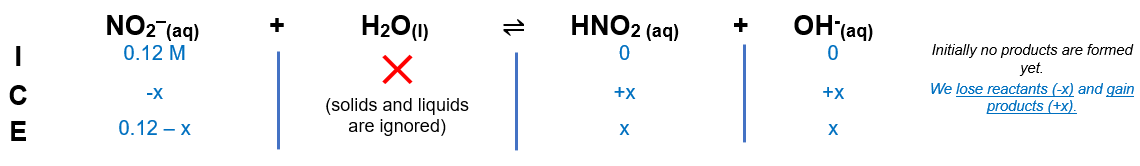# Problem: Calculate the pH of the following solution.a. 0.12 M KNO2

###### FREE Expert Solution

We are asked to Calculate the pH of 0.12 M KNO2

KNO2 → K+ + NO2-

• NO2- → conjugate base of HNO2

ICE Chart:Calculate x:

Kb is small so x is negligible in denominator.

[OH-] = x= 1.29 x10-6

Calculate pOH:

pOH = 5.89

89% (296 ratings)###### Problem Details

Calculate the pH of the following solution.

a. 0.12 M KNO2